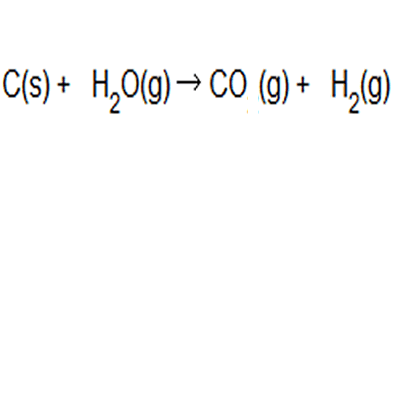Atom economy and percentage yield.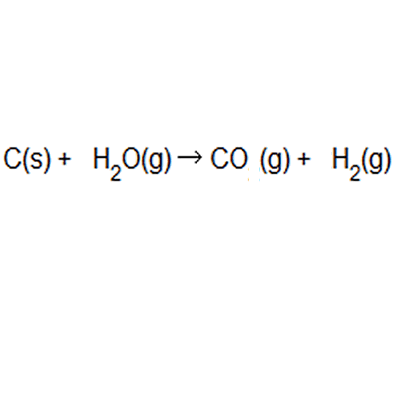Atom economy and percentage yield are indicators of how efficient a chemical reaction is. Atom economy is the second principle of green chemistry The ideal atom economy, known as 100% atom economy, for a chemical reaction is taken as the process where all reactant atoms are found in the desired product . In other words, atom economy is a calculation which measures "how much of the reactant atoms actually form the final product", the higher the atom economy the lower the amount of waste product formed.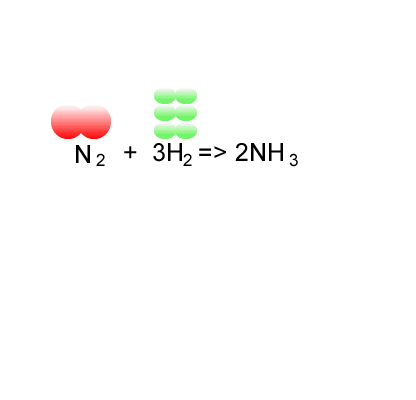This can be calculated from the balanced chemical equation prior to any reaction taking place. Atom economy does not take into account yield and does not allow for the fact that many industrial processes deliberately use excess of reactants to increase the yield of a required product. Where atom economy proves useful, however, is in comparing different reaction pathways to a desired product. Atom economy is the percentage of the total mass of reactants that successfully converted to the desired product.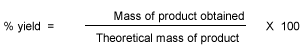Percentage yield is calculated from the mass of reactants and the mass of products.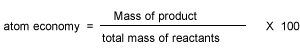Let's do some examples of simple reactions. Consider the combustion of methane to form carbon dioxide and water. CH4(g) + 2O2(g) => 2H2O(g) + CO2(g) In this particular reaction 32.0 grams of methane burns completely to produce 66.0 grams of water. What is the percentage yield? Step 1 Convert mass of methane into mol of methane => 32.0 /16.0 = 2.00 mol Step 2 Calculate the mol of water 2.00 mol of methane will produce according to the stoichiometry => 2 X 2.00 = 4.00 mol. Step 3 Calculate the theoretical mass of water produced => 4.00 X 18.0 = 72.0 grams Step 4 Calculate the % yield => (66.0/72.0) X 100 = 91.7% What is the atom economy of the reaction below in producing water? CH4(g) + 2O2(g) => 2H2O(g) + CO2(g) Step 1 Calculate the total mass of reactants as per equation. => 16.0 + 2 X 32.0 = 80.0 g Step 2 Calculate the mass of water as per equation => 2 X 18.0 = 36.0 g Step 3 Calculate the atom economy => (36.0 / 80.0 ) X 100 = 45.0%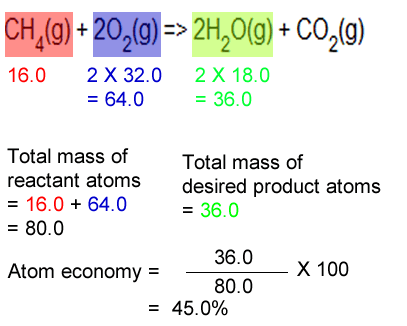Consider the synthesis of maleic anhydride from benzene as shown on the right. Calculate the atom economy for this reaction. Solution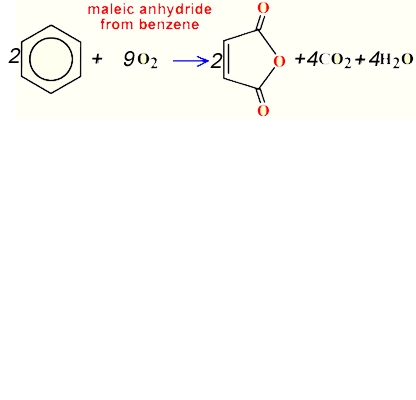Hydrogen is made by two complete processes. One involves reaction of steam and coal and the other involves reaction between methane and steam C(s) + 2H2O(g) → CO2(g) + 2H2(g) CH4(g) + H2O (g)→ CO(g) + 3H2(g) What is the atom economy for making hydrogen by reacting coal with steam according to the equation above? Solution What is the atom economy for making hydrogen by reacting methane with steam according to the equation above? Solution A low atom economy indicates that the reaction is efficient inefficient fast slow Methane gas can be produced through microbial breakdown of organic waste. Which reaction should be used to produce hydrogen gas? Give two reasons. SolutionConsider the formation of ammonia via the Haber process. Nitrogen is reacted with hydrogen to produce ammonia, according to the equation below. N2(g) + 3H2(g) => 2NH3(g) The hydrogen, however, comes from the reaction between methane and water according to the equation below. CH4(g) + H2O (g)→ CO(g) + 3H2(g) Calculate the atom economy for the formation of ammonia. SolutionHydrogen gas is produced from coal and steam in a controlled process to produce carbon monoxide and hydrogen gas. The equation to this chemical reaction is shown on the right. What is the atom of economy with respect to hydrogen gas production? Solution